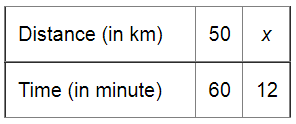# A car is travelling at the average speed of 50 km/hr.`
Question:

A car is travelling at the average speed of 50 km/hr. How much distance would it travel in 12 minutes?

Solution:Let the distance be $x \mathrm{~km}$.

If the time taken is less, the distance covered will also be less.

Therefore, it is a direct variation.

$50: x=60: 12$

$\Rightarrow \frac{50}{x}=\frac{60}{12}$

Applying cross muliplication, we get:

$x=\frac{50 \times 12}{60}$

$=10$

Thus, the required distance will be $10 \mathrm{~km}$.